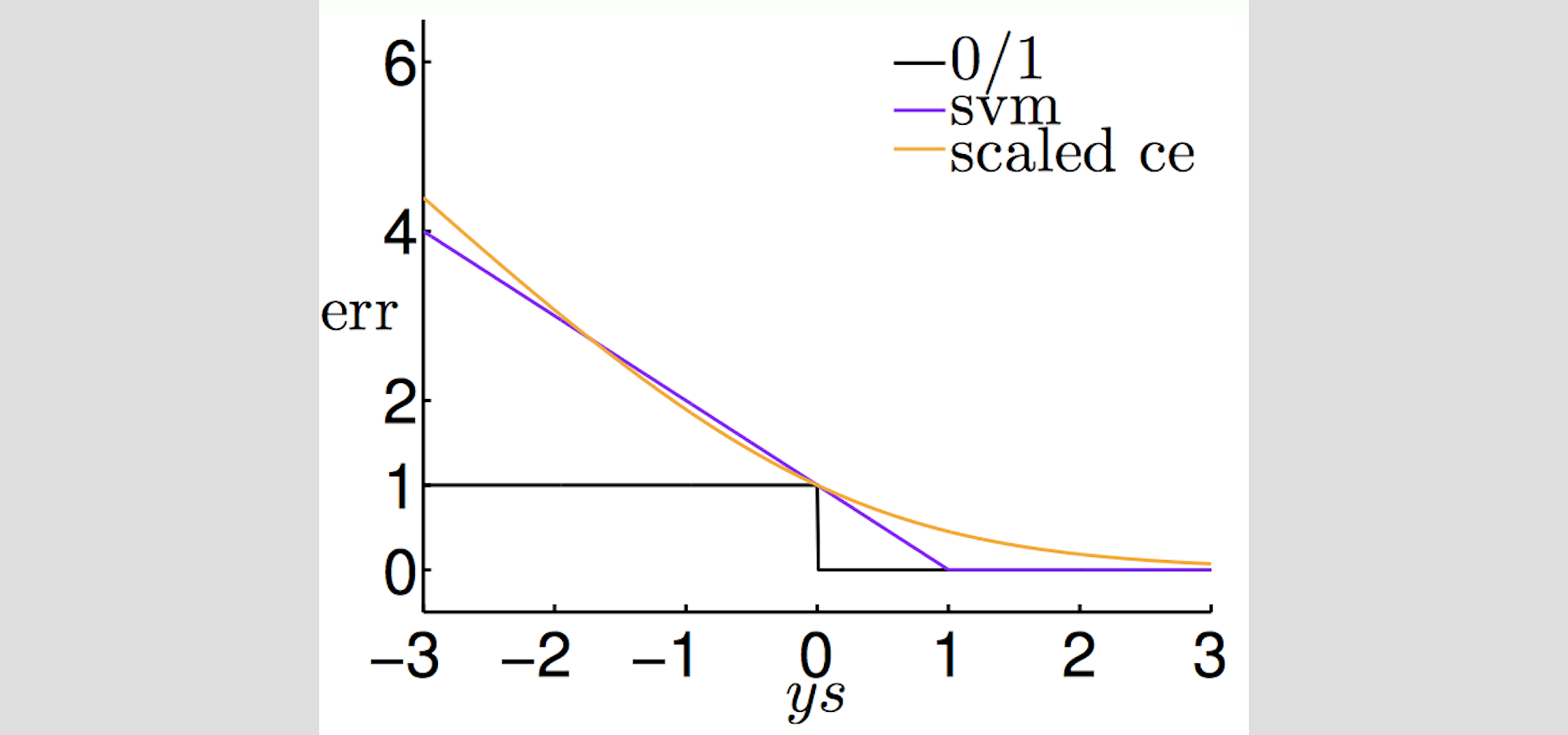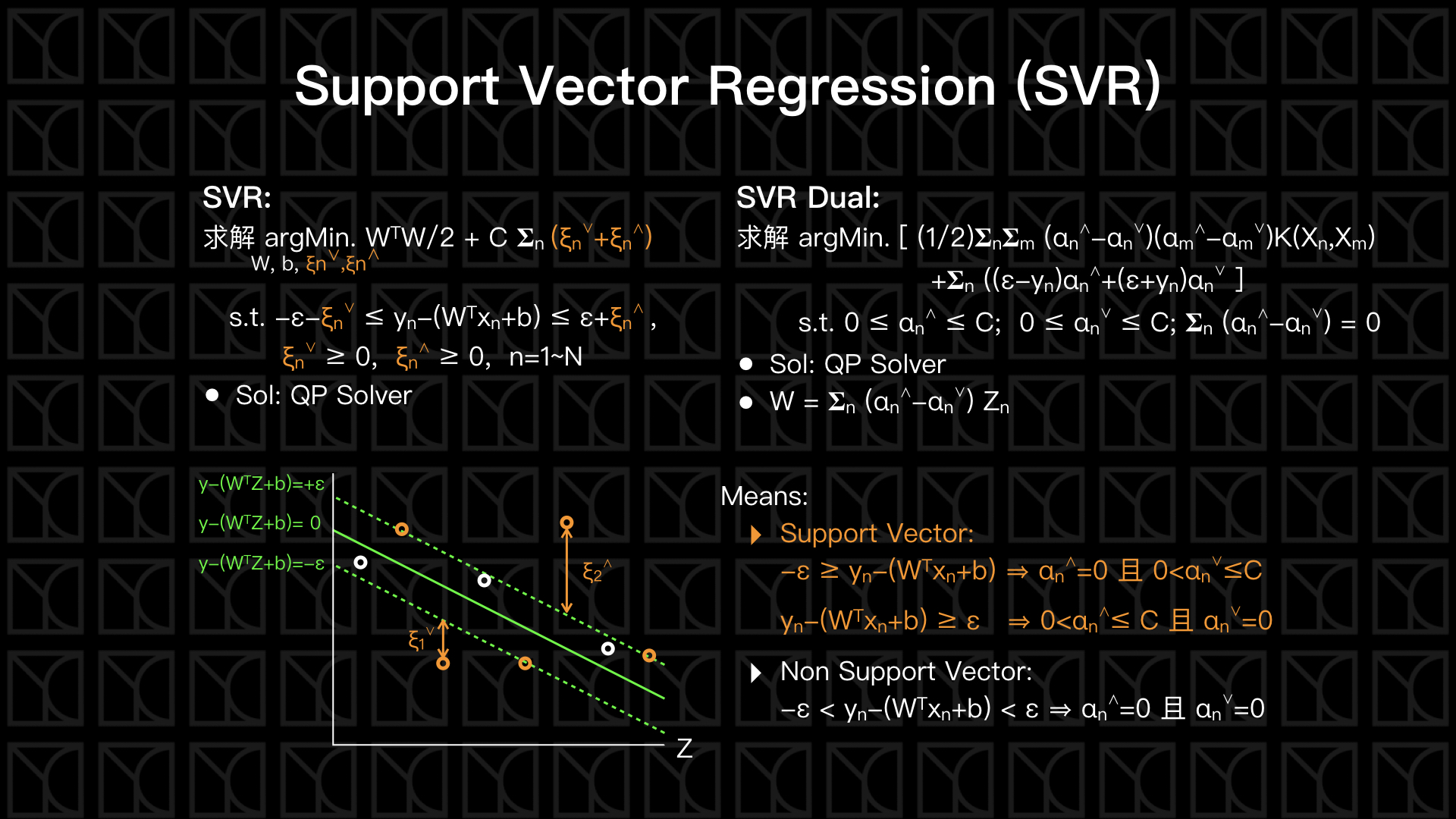# 機器學習技法 學習筆記 (3)：Kernel Regression

Posted on March 15, 2017 in AI.ML. View: 5,738

### Soft-Margin SVM其實很像L2 Regularized Logistic Regression

Soft-Margin SVM：

$$min. (W^{T}W/2) + C×𝚺_{n} ξ_{n}\ \ \ s.t.\ \ \ y_{n}×(W^{T}Z_{n}+b) ≥ 1-ξ_{n}\ and\ ξ_{n} ≥ 0,\ n=1\cdots N$$

Soft-Margin SVM：

$$min. C×𝚺_{n} Err_{hinge,n} + (W^{T}W/2)$$

L2 Regularized Logistic Regression：

$$min. (1/N)×𝚺_{n} Err_{ce,n} + (λ/N)×W^{T}W$$$$Err_{hinge,n}$$$$Err_{ce,n}$$是非常接近的，所以我們可以說做Soft-Margin SVM，很像是在做L2 Regularized Logistic Regression。

### 使用SVM做Logistic Regression：Probabilistic SVM

1. 使用Soft-Margin SVM解出切平面$$W_{SVM}^{T}Z+b_{SVM}=0$$，並將所有Data進一步的轉換到 $$Z'_{n}=W_{SVM}^{T}Z(X_{n})+b_{SVM}$$
2. 接下來用轉換後的結果$$\{Z'_{n},\ y_{n}\}$$做Logistic Regression得到係數A和B。
3. 最後的Target Function就是 $$g(x)=Θ(A\cdot (W_{SVM}^{T}Z(X_{n})+b_{SVM})+B)$$$$Θ$$為Logistic Function。

### Kernel Trick的真正精髓：Representer Theorem

Kernel Soft-Margin SVM：

$$0 ≤ α_{n} ≤ C;\ 𝚺_{n} α_{n}y_{n} = 0$$的限制條件下，求解$$min. [(1/2)𝚺_{n}𝚺_{m} α_{n}α_{m}y_{n}y_{m}K(X_{n},X_{m})-𝚺_{n} α_{n}]$$

$$W = 𝚺_{n} α_{n}y_{n}Z_{n}$$

$$b=y_{sv}-𝚺_{n} α_{n}y_{n}K(X_{n},X_{sv})$$### Kernel Logistic Regression

L2 Regularized Logistic Regression：

$$min. (1/N)×𝚺_{n} ln[1+exp(-y_{n}×(W^{T}Z_{n}))] + (λ/N)×W^{T}W$$

Kernel Logistic Regression:

$$min. (1/N)×𝚺_{n} ln[ 1+exp(-y_{n}×𝚺_{n} β_{n}K(X_{n},X)) ] + (λ/N)×𝚺_{n}𝚺_{m} β_{n}β_{m}K(X_{n},X_{m})$$

### Kernel Ridge Regression

Ridge Regression：

$$min. (1/N)×𝚺_{n} (y_{n}-W^{T}Z_{n})^{2} + (λ/N)×W^{T}W$$

Kernel Ridge Regression：

$$min. (1/N)×𝚺_{n} (y_{n}-𝚺_{m} β_{m}K(X_{n},X_{m}))^{2} + (λ/N)×𝚺_{n}𝚺_{m} β_{n}β_{m}K(X_{n},X_{m})$$

Kernel Ridge Regression：

$$min. E_{aug}$$

$$E_{aug}=(1/N)×(β^{T}K^{T}Kβ-2β^{T}K^{T}y+y^{T}y) + (λ/N)×β^{T}Kβ)$$

$$β^*=(λI+K)^{-1}y$$

Pseudo-Inverse：$$W=(X^{T}X)^{-1}X^{T}y$$

### Support Vector Regression (SVR)